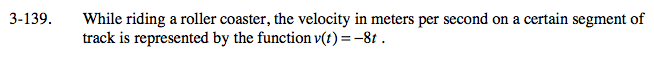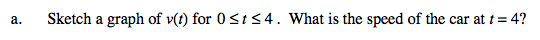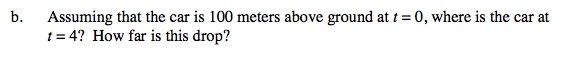### Home > CALC > Chapter 3 > Lesson 3.3.4 > Problem3-139

3-139.Recall that speed is the absolute value of velocity.

|v(4)| = |− 32| = 32
Don't forget the units!final position = initial position + displacement

intial position is 100 meters

Displacement is area between the velocity curve and x-axis. Notice that the area is negative (below the x-axis).

Total distance of the drop = area under a SPEED graph.